# PERSPECTIVE PROJECTION 3 D PROJECTION 3 D projection

• Slides: 34PERSPECTIVE PROJECTION…. .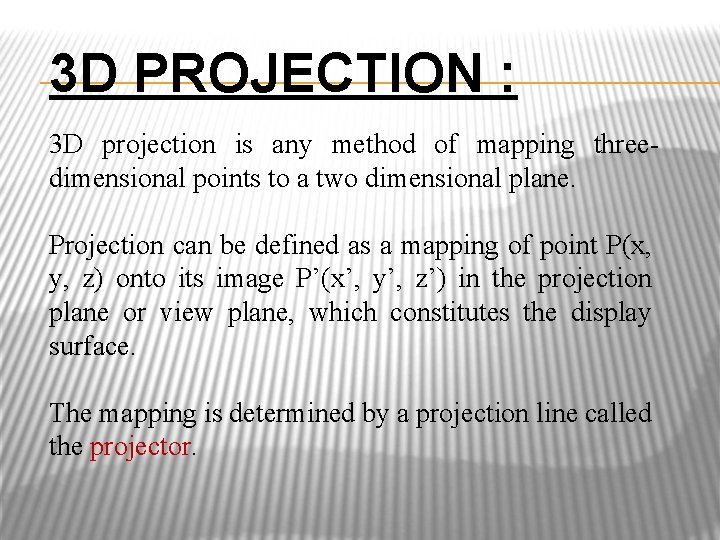3 D PROJECTION : 3 D projection is any method of mapping threedimensional points to a two dimensional plane. Projection can be defined as a mapping of point P(x, y, z) onto its image P’(x’, y’, z’) in the projection plane or view plane, which constitutes the display surface. The mapping is determined by a projection line called the projector.Why is it called Projection?Classification of Planar Geometric Projections 5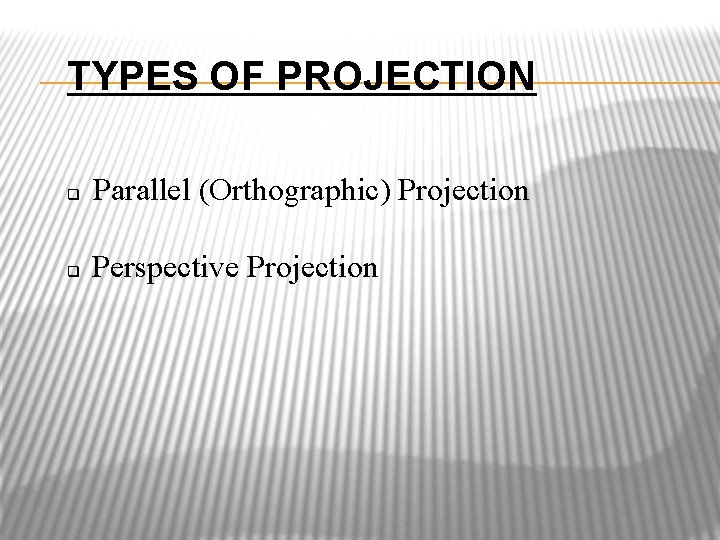TYPES OF PROJECTION Parallel (Orthographic) Projection Perspective Projection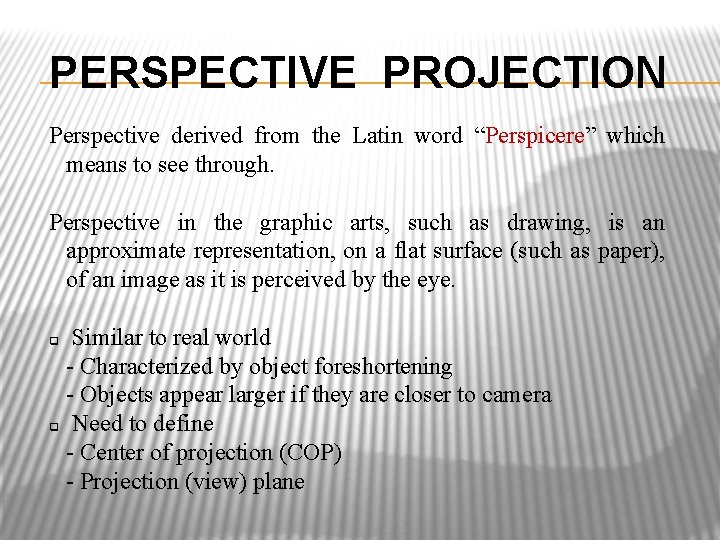PERSPECTIVE PROJECTION Perspective derived from the Latin word “Perspicere” which means to see through. Perspective in the graphic arts, such as drawing, is an approximate representation, on a flat surface (such as paper), of an image as it is perceived by the eye. Similar to real world - Characterized by object foreshortening - Objects appear larger if they are closer to camera Need to define - Center of projection (COP) - Projection (view) plane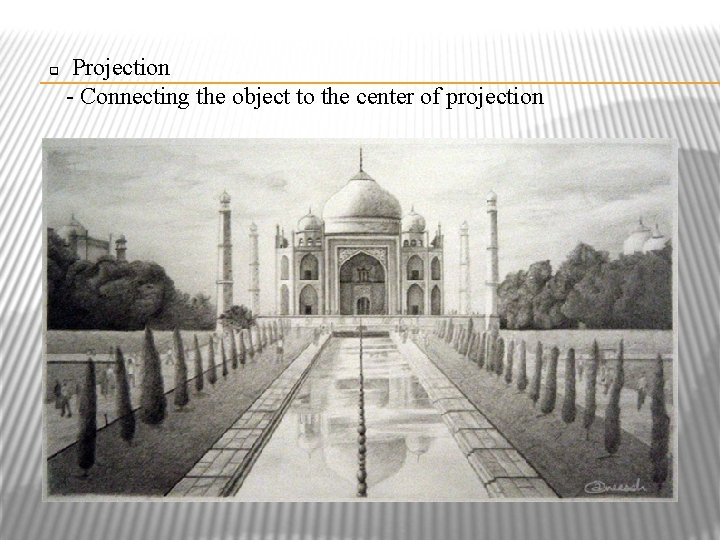Projection - Connecting the object to the center of projection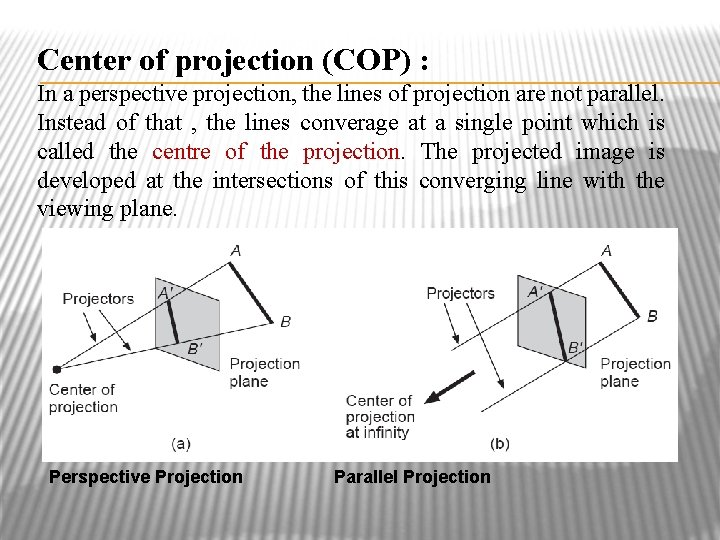Center of projection (COP) : In a perspective projection, the lines of projection are not parallel. Instead of that , the lines converage at a single point which is called the centre of the projection. The projected image is developed at the intersections of this converging line with the viewing plane. Perspective Projection Parallel Projection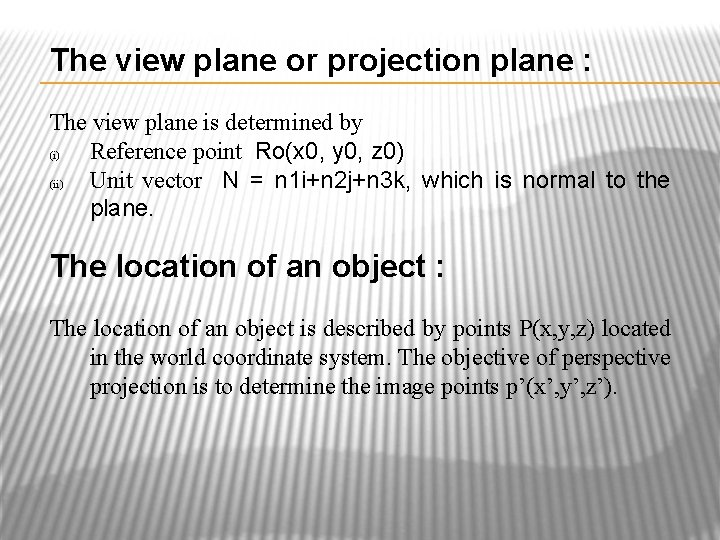The view plane or projection plane : The view plane is determined by (i) Reference point Ro(x 0, y 0, z 0) (ii) Unit vector N = n 1 i+n 2 j+n 3 k, which is normal to the plane. The location of an object : The location of an object is described by points P(x, y, z) located in the world coordinate system. The objective of perspective projection is to determine the image points p’(x’, y’, z’).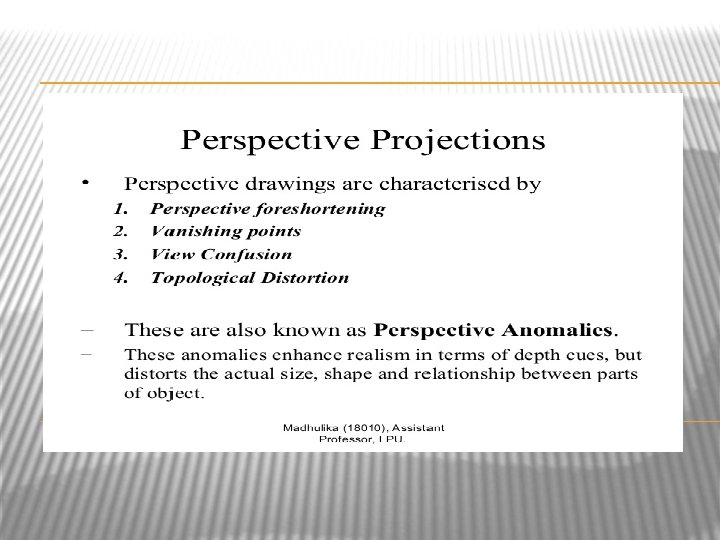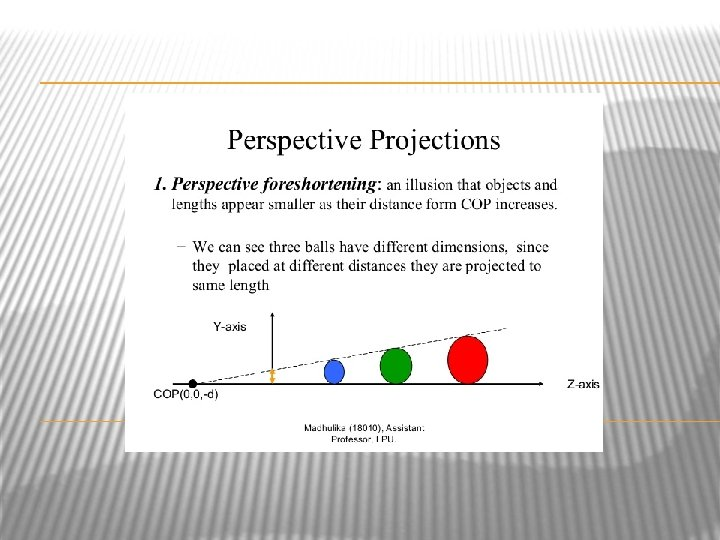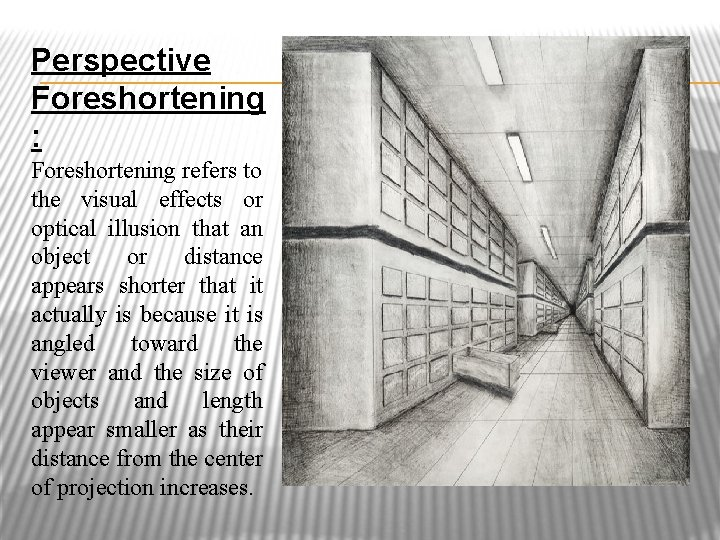Perspective Foreshortening : Foreshortening refers to the visual effects or optical illusion that an object or distance appears shorter that it actually is because it is angled toward the viewer and the size of objects and length appear smaller as their distance from the center of projection increases.Vanishing Points : Projection of lines that are not parallel to the view plane(i. e. lines that are not perpendicular to the view plane normal) appear to meet at some point on the view plane. This point is called the vanishing point.TYPES OF PERSPECTIVE PROJECTIONS : 1 - Point Perspective Projections 1.Examples of one-point perspective :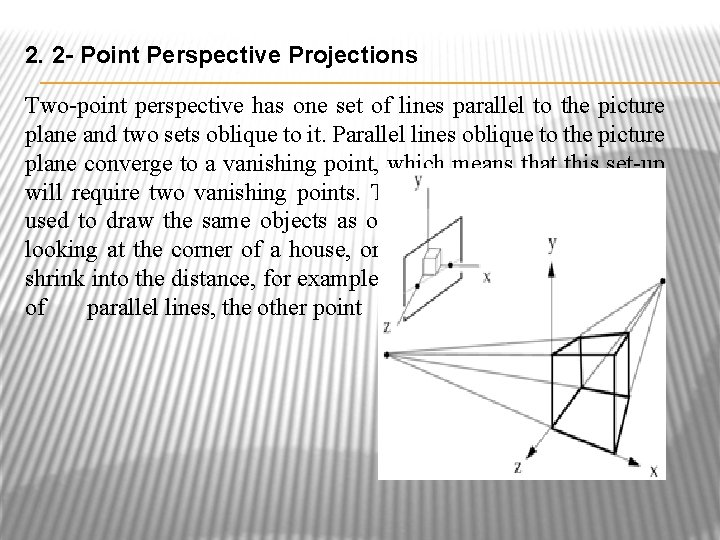2. 2 - Point Perspective Projections Two-point perspective has one set of lines parallel to the picture plane and two sets oblique to it. Parallel lines oblique to the picture plane converge to a vanishing point, which means that this set-up will require two vanishing points. Two-point perspective can be used to draw the same objects as one-point perspective, rotated: looking at the corner of a house, or looking at two forked roads shrink into the distance, for example. One point represents one set of parallel lines, the other point represents the other.Examples of two-point perspective :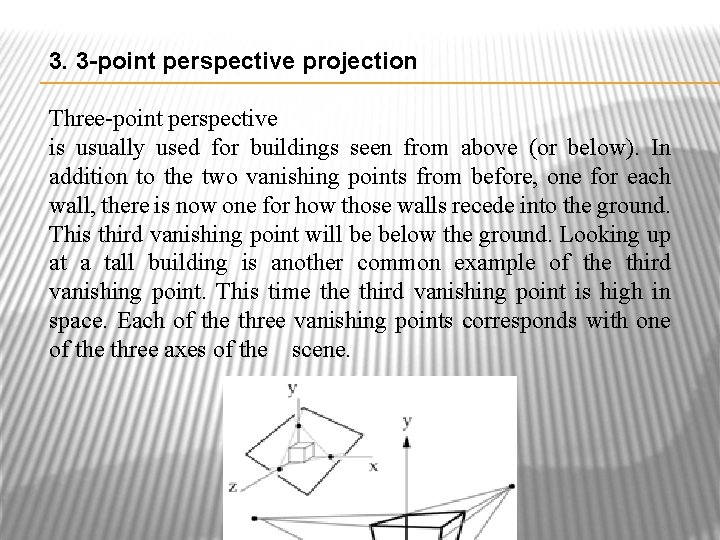3. 3 -point perspective projection Three-point perspective is usually used for buildings seen from above (or below). In addition to the two vanishing points from before, one for each wall, there is now one for how those walls recede into the ground. This third vanishing point will be below the ground. Looking up at a tall building is another common example of the third vanishing point. This time third vanishing point is high in space. Each of the three vanishing points corresponds with one of the three axes of the scene.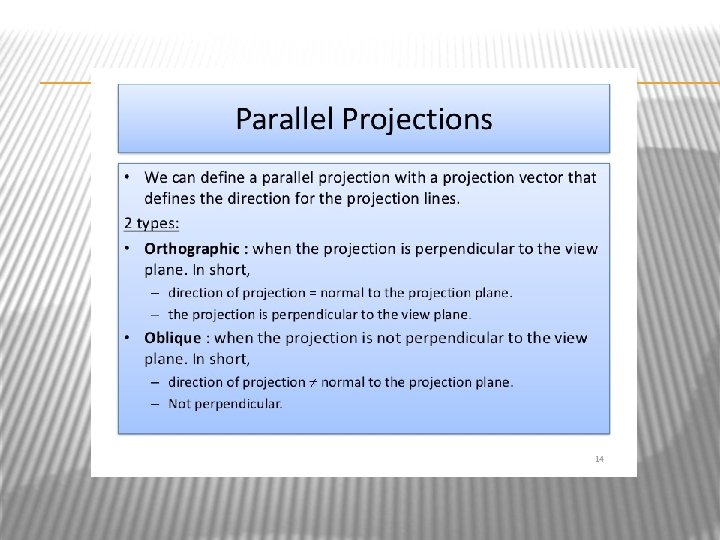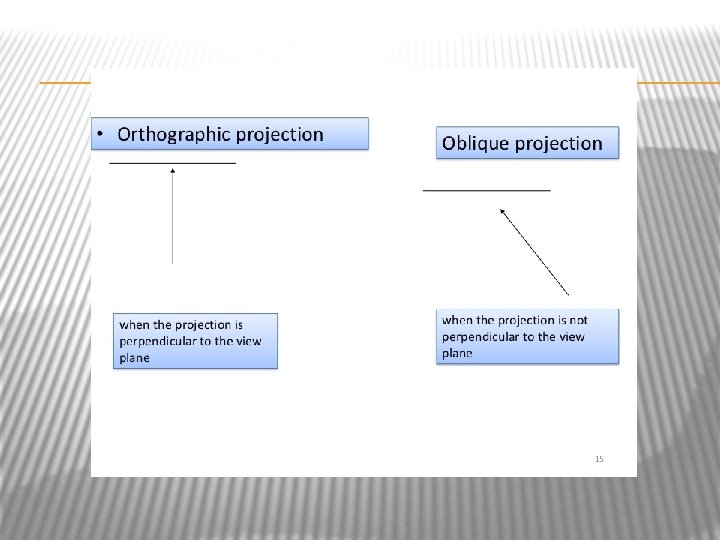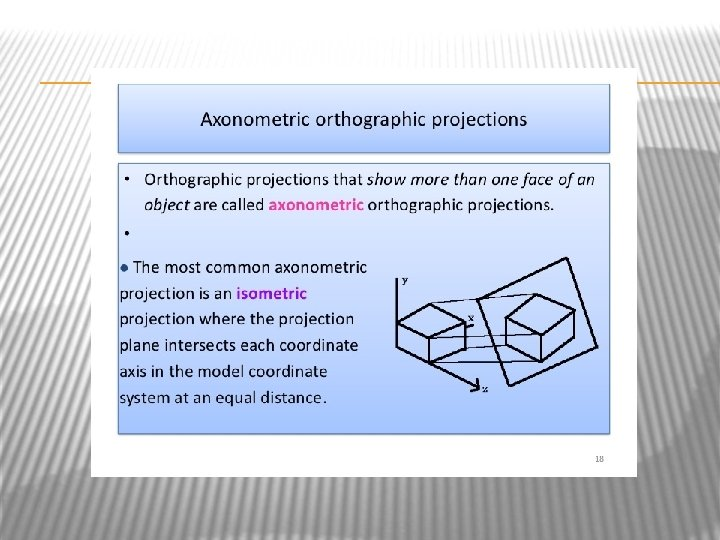Thanks… …